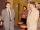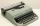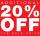Flowerbed

On the flowerbed was planted 280 flowers - pansies and crayons. During the first week wilts quarter of pansies and an eighth crayons, which is 20% of all flowers.

How many pansies were planted on the flowerbed?

Result

x =  168

Solution:x+y=280
1/4*x+1/8*y = 20/100*280

x+y = 280
50x+25y = 11200

x = 168
y = 112

Calculated by our linear equations calculator.

Leave us a comment of this math problem and its solution (i.e. if it is still somewhat unclear...):Be the first to comment!To solve this verbal math problem are needed these knowledge from mathematics:

Our percentage calculator will help you quickly calculate various typical tasks with percentages. Do you have a linear equation or system of equations and looking for its solution? Or do you have quadratic equation?

Next similar math problems:

1. CompetitorsIn the first round of slalom fell 15% of all competitors and in the second round another 10 racers. Together, 40% of all competitors fell. What was the total number of competitors?
2. Two workersTwo workers make 138 parts/items. The first one produces 30% more than the second. How many items will each produce?
3. Cinema ticketsCinema sold 180 tickets this Thursday, which is 20%. Monday 14%, Tuesday 6%, Wednesday 9%, Friday 24%, Saturday 12%, and Sunday 15%. How many tickets were sold per week?
4. TheatroTheatrical performance was attended by 480 spectators. Women were in the audience 40 more than men and children 60 less than half of adult spectators. How many men, women and children attended a theater performance?
5. Percents - easyHow many percent is 432 out of 434?
6. Belgium vs ItalyBelgium played a match with Italy and Belgium win by 2 goals. The match fell a total 6 goals. Determine the number of goals scored by Belgium and by Italy.
7. AssistantAssistant rewrote 15% of the entire manuscript in 12 hours. How many hours must still write to rewrite the rest of the manuscript?
8. PercentsHow many percents is 900 greater than the number 750?
9. The largerThe larger of two numbers is nine more than four times the smaller number. The sum of the two numbers is fifty-nine. Find the two numbers.
10. Spain vs USASpain lost to the US by 4 goals. In the match total fell 10 goals. How many goals gave the Spain and how the United States?
11. Jane classWhen asked how many students are in class, Jane said, if we increase the number of students in our class by hundred % and then add half the number of students, we get 100. How many students are in Jane's class?
12. ClassIn 7.C clss are 10 girls and 20 boys. Yesterday was missing 20% of girls and 50% boys. What percentage of students missing?
13. Boys and girlsThere are 48 children in the sports club, boys are 10 more than girls. How many girls go to the club?
14. Hotel roomsIn the 45 rooms, there were 169 guests, some rooms were three-bedrooms and some five-bedrooms. How many rooms were?
15. New refrigeratorNew refrigerator sells for 1024 USD, Monday will be 25% discount. How much USD will save, and what will be the price?
16. Percentage reductionReducing the unknown number by 19% get number 700.7 Determine unknown number.
17. 13 ticketsA short and long sightseeing tour is possible at the castle. Ticket for a short sightseeing circuit costs CZK 60, for a long touring circuit costs CZK 100. So far, 13 tickets have been sold for 1140 CZK. How much did you pay for tickets for a short tour?